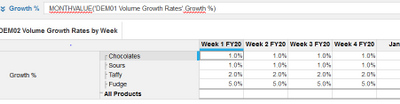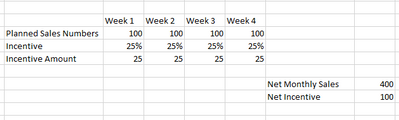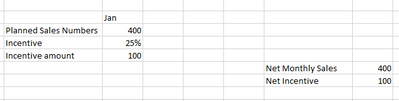# Level 2 - 2.3.8.4 ActivityI used the following formula to return the monthly value to weeks; MONTHVALUE('DEM01 Volume Growth Rates'.Growth %). I would have assumed that the monthly figure would be divided by 4 for each of the weeks. However, it is reflecting the same monthly value across all of the weeks.

Maybe i'm missing the intuition behind this. I know that the monthvalue is the correct formula to use, but not sure why it isn't returning the value I was hoping for.Tagged:

•The formula used in the module is absolutely correct. You are converting a monthly % value into a weekly % value that you would be using in the further weekly dimensioned modules. So you can understand this in the below way

The below is the Weekly Planned DataThe below is the same data but planned monthlySo the incentive percentage in the First table is the MONTHVALUE of the incentive Percentage of the second table

I hope you understand the logic

~Anand